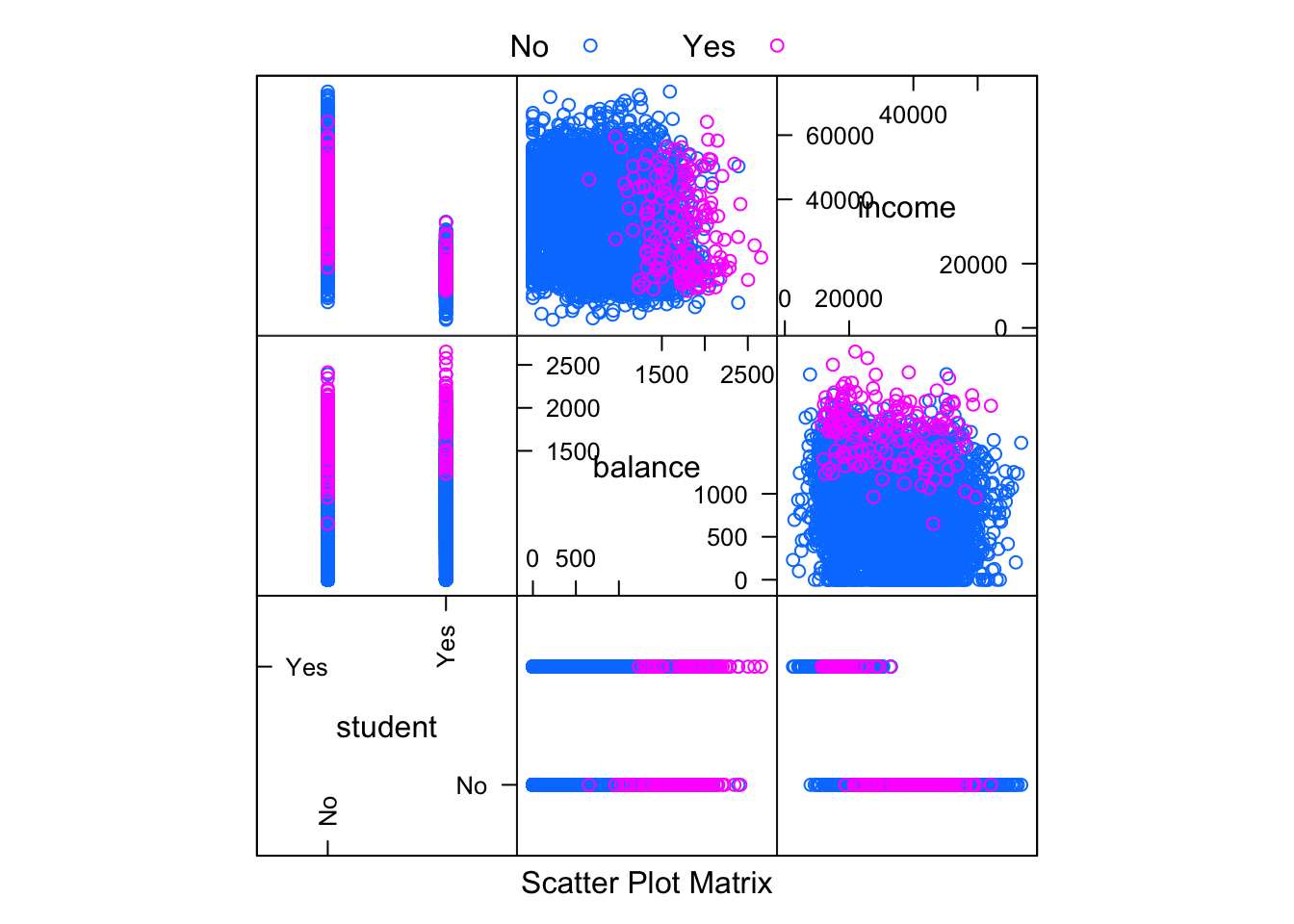The notes on using KNN for Classification use the `knn()` function from the `class` package. This implementation has several disadvantages:

• Inability to use formula syntax.
• Inability to use the fit then predict setup. Must immediately supply observations to make predictions.
• Only accepts numeric predictors.
• Only returns the predicted probability of the majority class.

The last issue is a serious limitation. This makes creating a binary classifier with a cutoff other than 0.5 extremely difficult.

To fix these issues, we will use the `knn3()` function from the `caret` package. It essentially works the same way as `knnreg()` from `caret`, but performs classification instead of regression. Because it is performing classification, we need to understand how it returns predicted probabilities.

# Packages

We’ll need the `ISLR` package for the data, and the `caret` package for model fitting.

``````library(ISLR)
library(caret)``````

# `Default` Data

``````set.seed(42)
default_idx = sample(nrow(Default), 5000)
default_trn = Default[default_idx, ]
default_tst = Default[-default_idx, ]``````

Unlike the notes, we do not need to coerce the student variable to be a numeric `0` / `1` variable, `knn3()` will take care of this for us.# KNN Model

``knn_mod = knn3(default ~ ., data = default_trn, k = 25)``

Here we see familiar syntax, which is practically identical to that of `knnreg()`.

``knn_mod``
``````## 25-nearest neighbor model
## Training set outcome distribution:
##
##   No  Yes
## 4832  168``````

We take a quick look at how the function is preprocessing the predictor data. (It’s using one-hot encoding of the factor variable `student`.)

``head(knn_mod\$learn\$X)``
``````##      studentYes   balance   income
## 9149          0  650.2901 44358.65
## 9370          0 1815.1741 23648.41
## 2861          0 1035.5529 29423.23
## 8302          1  193.7198 18002.55
## 6415          0  262.7913 28974.75
## 5189          1  576.0650 13536.61``````

# Using `predict()`

Calling predict on an object returned by `knn3()` allows for two possibilities, predicted probabilities, or classifications.

``````# return classifications (classifying to majority class)
head(predict(knn_mod, default_tst, type = "class"), n = 10)``````
``````##   No No No No No No No No No No
## Levels: No Yes``````

Here we are returning classifications for the first 10 observations in the test set.

``````# return predicted probabilities
head(predict(knn_mod, default_tst, type = "prob"), n = 10)``````
``````##         No  Yes
##  [1,] 1.00 0.00
##  [2,] 1.00 0.00
##  [3,] 1.00 0.00
##  [4,] 1.00 0.00
##  [5,] 1.00 0.00
##  [6,] 0.88 0.12
##  [7,] 1.00 0.00
##  [8,] 1.00 0.00
##  [9,] 0.96 0.04
## [10,] 1.00 0.00``````

Here we are returning predicted probabilities for the first 10 observations in the test set. Notice that we obtain probabilities for both possible classes, stored in columns.

# Formula Syntax

Here we utilize formula syntax for easy scaling of the numeric predictors.

``````knn_mod_scale = knn3(default ~ scale(income) + scale(balance) + student,
data = default_trn, k = 25)
``````##      scale(income) scale(balance) studentYes
## 9149     0.7878907     -0.3951736          0
## 9370    -0.7638954      2.0039814          0
## 2861    -0.3311972      0.3983004          0
## 8302    -1.1869314     -1.3355103          1
## 6415    -0.3648012     -1.1932528          0
## 5189    -1.5215573     -0.5480451          1``````

# Model Evaluation

First we obtain and store classifications for both models using the test set. (Unscaled and scaled. Both using `k = 25`. Note we didn’t tune `k` here, but we should in practice!)

``````tst_pred_un = predict(knn_mod, default_tst, type = "class")
tst_pred_sc = predict(knn_mod_scale, default_tst, type = "class")``````
``````calc_class_err = function(actual, predicted) {
mean(actual != predicted)
}``````

Then we compare their classification error rates.

``calc_class_err(default_tst\$default, tst_pred_un)``
``##  0.0326``
``calc_class_err(default_tst\$default, tst_pred_sc)``
``##  0.027``

It seems that in this case, scales performs slightly better. We investigate this model further with a confusion matrix and additional metrics.

``````# let caret calculate evaluation metrics
sc_results = confusionMatrix(table(predicted = tst_pred_sc,
actual = default_tst\$default),
positive = "Yes")``````

Be sure to declare the “positive” class when using the `confusionMatrix()` function, else, you might flip sensitivity and specificity.

``````# confusion matrix
sc_results\$table``````
``````##          actual
## predicted   No  Yes
##       No  4819  119
##       Yes   16   46``````
``sc_results\$overall["Accuracy"]``
``````## Accuracy
##    0.973``````
``````c(sc_results\$byClass["Sensitivity"],
sc_results\$byClass["Specificity"],
sc_results\$byClass["Prevalence"])``````
``````## Sensitivity Specificity  Prevalence
##   0.2787879   0.9966908   0.0330000``````

# `iris` Data

KNN can also be used when the response has more than two categories.

``````set.seed(430)
iris_obs = nrow(iris)
iris_idx = sample(iris_obs, size = trunc(0.50 * iris_obs))
iris_trn = iris[iris_idx, ]
iris_tst = iris[-iris_idx, ]``````